# Heaps

Note: The term "heap" is used in a completely different way in Chapter 16.

A heap is a binary tree of Comparables with the following special properties:

• The value at each node is less than or equal to the values at the children of that node.
• The tree is perfect or close to perfect. It might be missing some nodes on the right end of the bottom level.

An example is shown in Figure 14-1.

Figure 14-1. In a heap, each node is less than or equal to its children. It follows that the smallest element is at the root. On the other hand, a node may be lower in the tree than a smaller cousin (compare 6 and 8).

(This item is displayed on page 370 in the print version)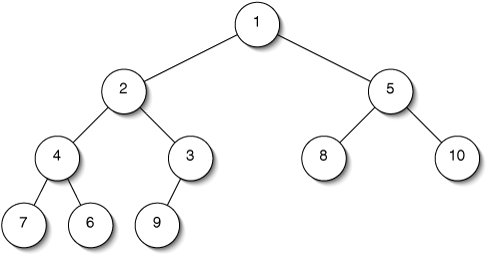We could implement a heap using BinaryNodes (Section 10.1), but there is a more efficient representation. The requirement that a heap is "perfect or close to perfect" lets us use a contiguous representation somewhat analogous to the representation of multidimensional arrays from Section 12.3. We use an ArrayList, with the root at position 0, its children in the next two positions, their children in the next four, and so on (Figure 14-2). The constraint on the shape of the tree ensures that there will be no gaps in this representation.

Figure 14-2. A heap can be represented using an ArrayList. The levels of the tree (highlighted by shading) are represented, one after another, in the array.This representation is more space-efficient than a linked one, but how do we find the relatives of a node? We only have to do a little arithmetic.

The left child of the node at index i is at index 2i + 1. Take a moment to verify this in Figure 14-2. The right child is at index 2i + 2. The parent is at index: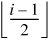The basic outline of the Heap class, including methods to find relatives, is given in Figure 14-3.

Figure 14-3. Easy parts of the Heap class.

 ``` 1 /** 2 * A nearly perfect tree where nodes are <= their children. 3 * Can be used as a priority queue or for heapsort. 4 */ 5 public class Heap> { 6 7 /** Contiguous representation of the tree. */ 8 private ArrayList data; 9 10 /** The tree is initially empty. */ 11 public Heap() { 12 data = new ArrayList(); 13 } 14 15 /** Return true if this Heap is empty. */ 16 public boolean isEmpty() { 17 return data.isEmpty(); 18 } 19 20 /** Return the index of the left child of the node at index. */ 21 protected static int leftChildIndex(int index) { 22 return (2 * index) + 1; 23 } 24 25 /** Return the index of the parent of the node at index. */ 26 protected static int parentIndex(int index) { 27 return (index - 1) / 2; 28 } 29 30 /** Return the index of the right child of the node at index. */ 31 protected static int rightChildIndex(int index) { 32 return (2 * index) + 2; 33 } 34 35} ```

Priority Queues

A heap is a good data structure for implementing a priority queue. Recall that when we remove something from a regular queue (Section 4.4), we get the oldest element. In a priority queue, on the other hand, we get the smallest element. It's easy to find the smallest element in a heap: it's always at index 0.

If we want to add something to a priority queue, we start by tacking it onto the end of the ArrayList. We can't stop there, because the tree represented by this list might not be a valid heap any more. Specifically, the new element might be smaller than its parent. We fix this problem by filtering the offending element up toward the root until it is in a valid position (Figure 14-4).

Figure 14-4. Filtering up in a heap. When a new node is added (top), it is compared with its parent. If it is smaller than its parent, they are swapped (middle). This continues until the new element moves up to its proper place (bottom).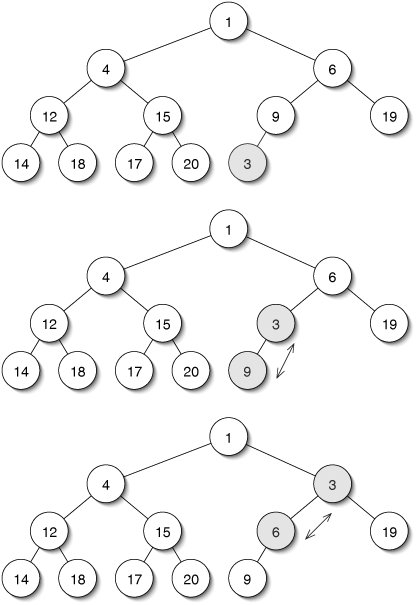Even in the worst case, this takes time proportional to the height of the tree. Since the tree is perfect or close to perfect, this is in O(log n). The code is given in Figure 14-5.

Figure 14-5. Adding a new element to a priority queue involves filtering it up to its proper place in the heap.

 ``` 1 /** Add a new element, maintaining the heap properties. */ 2 public void add(E target) { 3 data.add(target); 4 filterUp(data.size() - 1); 5 } 6 7 /** Move the element at index up to restore the heap properties. */ 8 protected void filterUp(int index) { 9 int parent = parentIndex(index); 10 while (parent >= 0) { 11 if (data.get(index).compareTo(data.get(parent)) < 0) { 12 swap(index, parent); 13 index = parent; 14 parent = parentIndex(index); 15 } else { 16 return; 17 } 18 } 19 } 20 21 /** Swap the elements at indices i and j. */ 22 protected void swap(int i, int j) { 23 E temp = data.get(i); 24 data.set(i, data.get(j)); 25 data.set(j, temp); 26 } ```

Removing an element from a priority queue is only slightly more complicated (Figure 14-6). We remember the element at index 0 so we can return it later. The element in the last position is then copied over the root and filtered down until it is in a legitimate position. If both children are smaller than their parent, we swap the parent with the smaller of the two.

Figure 14-6. When the root is removed from a heap (top), it is replaced by the last element (second from top). This element is then filtered down to a legitimate position.

(This item is displayed on page 374 in the print version)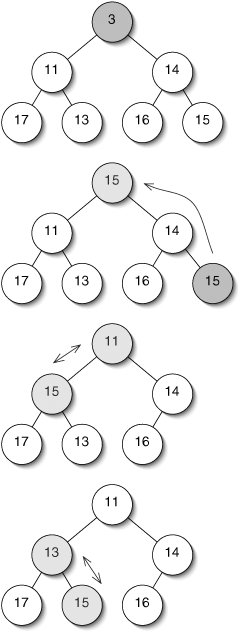This operation also takes time in O(log n). The code (Figure 14-7) is somewhat long because of the three-way comparison between a node and its children.

Heapsort

Heaps are also useful for a sorting algorithm called heapsort. The algorithm begins by copying the data to be sorted into a heap. The filterDown() method is then invoked several times to make the heap valid. Finally, we remove elements from the heap one at a time. Since each removal from the heap returns the smallest remaining element, they are removed in increasing order.

Since we've already laid most of the groundwork, the code for the heapsort() method is remarkably short (Figure 14-8). Lines 79 copy unsortedData, taking time in Q(n). Line 11, which takes time in O(log n), is run no more than n times, for a total in O(n log n). The same is true of line 20. The total worst-case time for heapsort is therefore in O(n log n). Heapsort is a comparison sort (Section 12.4), so we can conclude that the worst-case time is precisely in Q(n log n).

Figure 14-7. Code for removing an element from a priority queue.

 ``` 1 /** Move the element at index down to restore heap properties. */ 2 protected void filterDown(int index) { 3 while (index < data.size()) { 4 int left = leftChildIndex(index); 5 int right = rightChildIndex(index); 6 int smallest = index; 7 if ((left < data.size()) 8 && (data.get(left).compareTo(data.get(smallest)) < 0)) { 9 smallest = left; 10 } 11 if ((right < data.size()) 12 && (data.get(right).compareTo(data.get(smallest)) < 0)) { 13 smallest = right; 14 } 15 if (index == smallest) { 16 return; 17 } 18 swap(index, smallest); 19 index = smallest; 20 } 21 } 22 23 /** Remove and return the smallest element in the Heap. */ 24 public E remove() { 25 E result = data.get(0); 26 E lastElement = data.remove(data.size() - 1); 27 if (data.size() > 0) { 28 data.set(0, lastElement); 29 } 30 filterDown(0); 31 return result; 32 } ```

Figure 14-8. Heapsort. The constructor on lines 113 is a second, overloaded constructor for the Heap class; the other was in Figure 14-3.

 1 /** 2 * Copy the elements of unsortedData into the tree, then 3 * rearrange them to make it a heap. 4 */ 5 protected Heap(ArrayList unsortedData) { 6 data = new ArrayList(); 7 for (E e : unsortedData) { 8 data.add(e); 9 } 10 for (int i = (data.size() / 2) - 1; i >= 0; i--) { 11 filterDown(i); 12 } 13 } 14 15 /** Sort data. */ 16 public static > void 17 heapsort(ArrayList data) { 18 Heap heap = new Heap(data); 19 for (int i = 0; i < data.size(); i++) { 20 data.set(i, heap.remove()); 21 } 22 }

The type parameter specified between static and void on line 16 is necessary because heapsort() is a static method; it is not associated with a particular instance of Heap, although it creates one on line 18. Since the type parameter E at the beginning of the Heap class might stand for different things in different instances of Heap, a static method like heapsort() has to specify a new type parameter.

Java's PriorityQueue Class

The java.util package contains a PriorityQueue class. The comments for Java's Queue interface are carefully worded to encompass both FIFO queues (Section 4.4) and priority queues. The LinkedList and PriorityQueue classes therefore both extend this interface (Figure 14-9).

Figure 14-9. The java.util.Queue interface is implemented by both LinkedList and PriorityQueue. The add() and remove() methods inherited from Collection each return a boolean value indicating whether the operation succeeded.Exercises

14.4

 14.1 Can a tree ever be both a heap and a binary search tree? If so, give an example. If not, explain why not. 14.2 Write methods leftSiblingIndex() and rightSiblingIndex() for the Heap class. 14.3 On lines 1012 of Figure 14-8, filterDown() is invoked only on the firstelements of data. How can we be sure that the heap is valid at the end of this? Why does i decrease to 0 instead of increasing from 0? Java's built-in TreeSet class uses time in O(log n) for insertion and deletion. It seems that we could build a Q(n log n) sorting algorithm by simply inserting all of the data into a TreeSet, then traversing the TreeSet inorder (Figure 14-10). The running-time analysis is correct, but this algorithm fails to sort some ArrayLists. Why? (Hint: Think about the definition of a Set.

Figure 14-10. Code for Exercise 14.4.

 ```1 /** Sort data. */ 2 public static > void 3 sort(java.util.ArrayList data) { 4 java.util.TreeSet tree = new java.util.TreeSet(data); 5 data.clear(); 6 for (E item : tree) { 7 data.add(item); 8 } 9 } ```

### Disjoint Set Clusters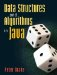Data Structures and Algorithms in Java
ISBN: 0131469142
EAN: 2147483647
Year: 2004
Pages: 216
Authors: Peter Drake